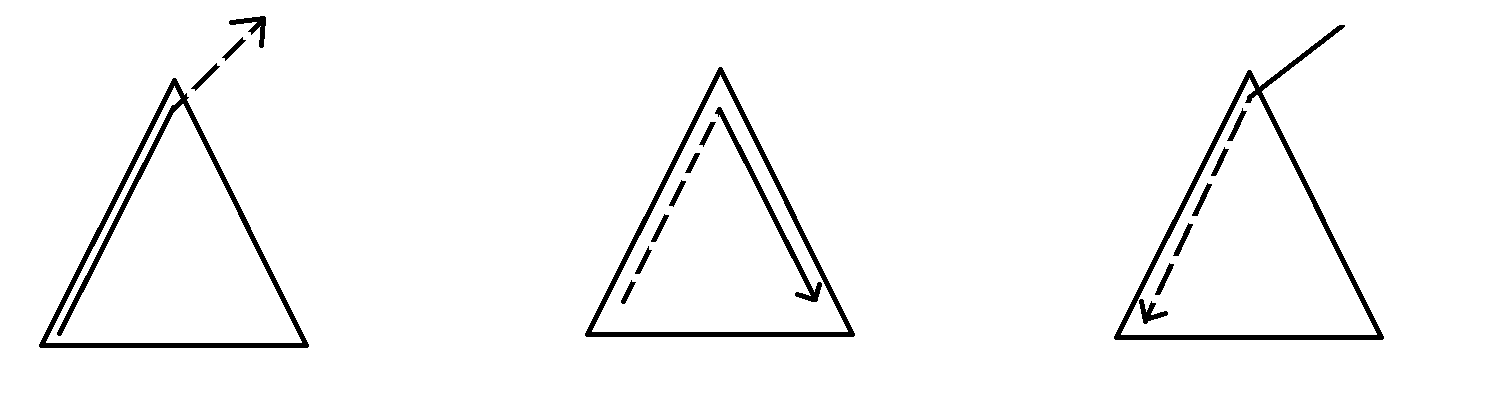# arrow_back树形dp共11篇文章zc
2020-10-21 16:41:02

## 解法二zc
2019-12-21 19:47:00zc
2019-12-21 19:47:00

1. 直接通电 : q_i

2. 被子节点通电 :

(1-q_i) \times P_{to}(\text{子节点}) \times e_i.w(\text{连接的边导电的概率})

P_x= P_x' (1-P_x')*(P_{to}*e_i.w)(P_x'指根节点除去当前子节点的贡献的答案)

(这里有个坑点:当1-(P_{to} \times e_i.w) = 0时这个式子就没有意义了)

\therefore P_{to} = P_{to} + (1-P_{to}) \times P_x' \times e_i.wzc
2019-12-21 19:47:00

f[i][j]为根为i的子树用了j条边,最多保留多少,

l,r表示左右节点,lw,rw表示连接左右儿子的边的权值分别为多少

f[i][j]=\max(lw+f[l][j-1],rw+f[r][j-1])

f[i][j]=\max(lw+f[l][k-1],rw+f[r][j-k-1])zc
2019-12-21 19:47:00

考虑 up:

s_x为点x子树中所有点到x的距离之和

S_x = \sum (s_{to}+siz_{to})

考虑 down:

s_{to} = s_{to} + (s_x-s_{to}-siz_{to}) + n - siz_{to}zc
2019-12-21 19:47:00

tarjan找出强连通分量之后树形dpzc
2019-12-21 19:47:00

## 解法2:zc
2019-12-21 19:47:00

f[i][j]表示在i的子树中,i所在的连体块大小为j\frac{\text{最大得分}}{j}

f[i][j] = \max(f[i][k] \times f[to][j-k])

f[i] = \max(f[i],f[i][j] \times j)zc
2019-12-21 19:47:001. x子树内\rightarrow x \rightarrow x子树外(包括x)

2. x子树内(包括x)\rightarrow x \rightarrow x子树内

3. x子树外\rightarrow x \rightarrow x子树内

1. ans += V_x \times (f_x-g_x) \times (n-siz_x+1)(可以在x的子树中随机选一个往子树外面走(可以指走到x))

2. ans += V_x \times (g_{to}-f_{to}) \times (siz_x-siz_{to}-1)

ans+= V_x \times (siz_x-1)(可以从x直接向下走)

3. 我们需要记录x子树外与x的距离为偶数和与x的距离为奇数的点的个数,分别记作uf_x,ug_x

我们可以通过bfs从上到下来处理,那么

uf_x=ug_{fa_x}+g_{fa_x}-f_x

ug_x=uf_{fa_x}+f_{fa_x}-g_x

ans += V_x \times (uf_x-ug_x) \times siz_xzc
2019-12-21 19:47:00

f[i][j]表示在i的子树中与i距离为j的点数

g[i][j]表示以i为根的子树中,点对(x,y)满足x,ylca(x,y)距离都是d,并且lca(x,y)i的距离为d-j的点对数

g[i][j] = \sum g[to][j+1]

ans = \sum_{i=1}^n (g[i]+g[i][j] \times f[to][j-1])

n \le 100000

Search
search